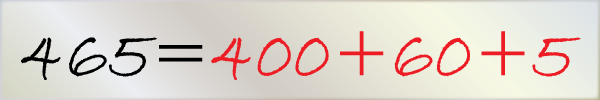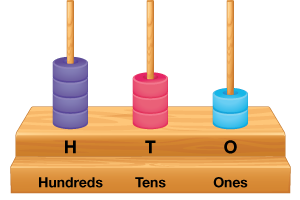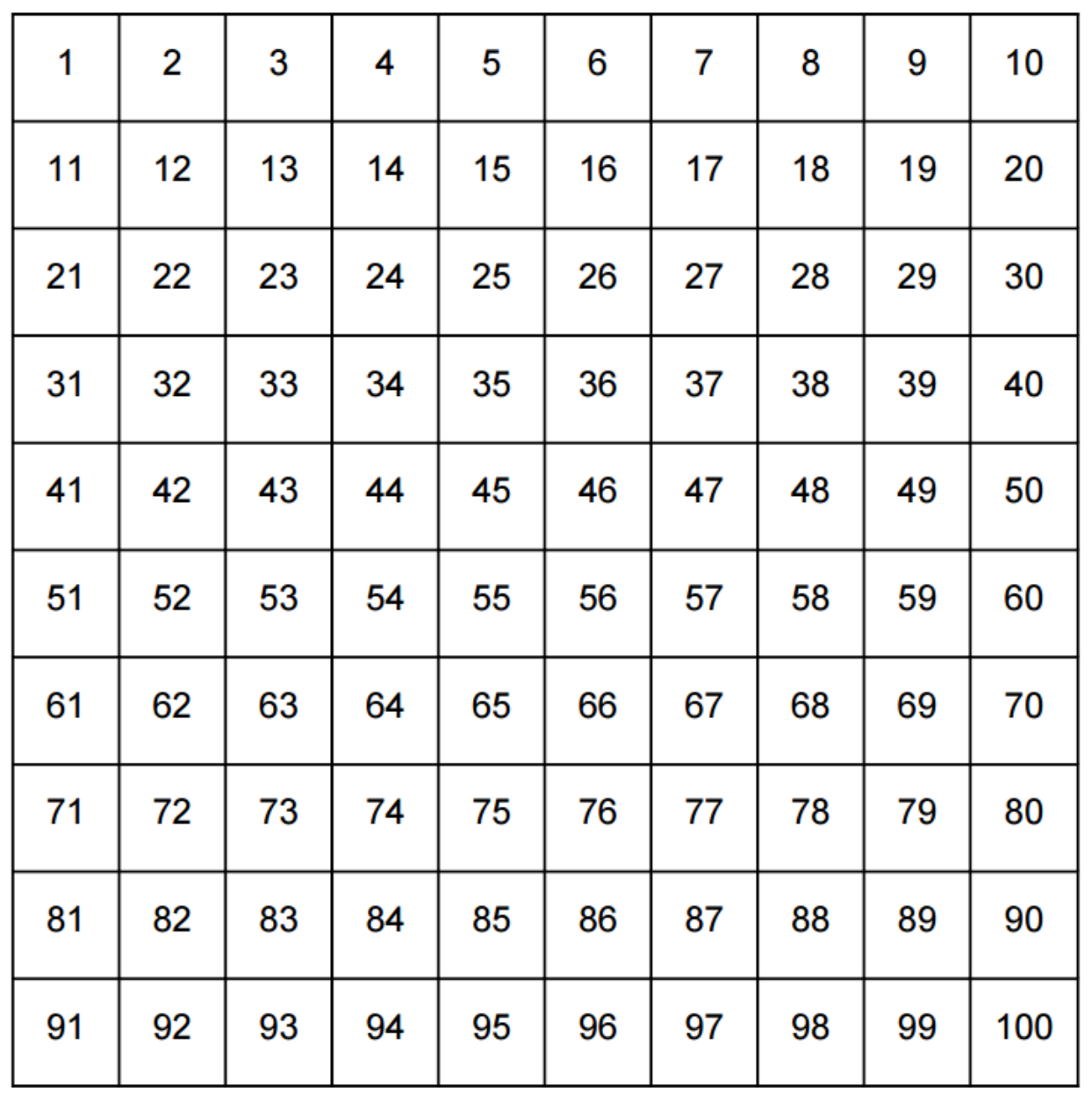In the verge of coronavirus pandemic, we are providing FREE access to our entire Online Curriculum to ensure Learning Doesn't STOP!

# Numbers up to 3-Digits

Go back to  'Numbers and Place values'

## Introduction to Numbers up to 3-Digits

We have already seen what $$2$$-digit numbers are. The first $$2$$-digit number is $$10,$$ and the last one is $$99,$$ so there are only $$90$$ two digit numbers possible. What would three digit numbers then be? In the same way, we can define three digit numbers as those which have digits in three place values – Units, Tens and Hundreds. These are also formed by combining any three single digit numbers.## The Big Idea: Numbers up to 3-Digits

### A simple idea: The Place Value of Numbers

We spoke earlier about how you can buy ice cream for the guests in your house. When the number of guests was in single digits, you bought ice cream cups (units). But when the requirement increased to a number more than $$10,$$ then you ordered the ice cream in a combination of tubs (equivalent to $$10$$ ice cream cups) and cups.

Now let us take this a step further, and go to the next higher power of $$10,$$ which is $$100.$$ So what would happen if your school was going on a picnic and you were put in charge of ordering ice creams for all $$243$$ participants, including teachers and students? You would first ask the shopkeeper if he has any bigger cases, and he would inform you that they do have a large carton which is equal to ten tubs. Since you already know that each tub is equivalent to ten cups, therefore

\begin{align} \text { Carton } & = 10 \text { tubs } \\ & = \mathrm { tub } + \mathrm { tub } + \mathrm { tub } + \mathrm { tub } + \mathrm { tub } + \mathrm { tub } + \mathrm { tub } + \mathrm { tub } + \mathrm { tub } \\ & = 10 \mathrm { cups } + 10 \mathrm { cups } + \ldots \ldots .10 \text { times } \\ & = 100 \mathrm { cups } \end{align}

Now, for $$243$$ participants, here are how the $$3$$ digits are placed in respective values:

\begin{align}& { 2 \text { in hundreds place } } \\& { 4 \text { in tens place } } \\& { 3 \text { in units place } } \\& { 243 = ( 2 \times 100 ) + ( 4 \times 10 ) + ( 3 \times 1 ) } \\& {\quad \;\;\; = 2 \text { cartons } + 4 \text { tubs } + 3 \text { cups } } \end{align}

So you would order $$2$$ cartons for $$200$$ cups, $$4$$ tubs for $$40$$ cups and $$3$$ cups.

### Assigning Place Values

Every three digit number’s value can be found by seeing which place value each digit has. Let us consider the same number $$243$$ as we saw in the ice cream example. We need to write the number as the sum of the products of each digit and its place value.

The first digit at the rightmost position is said to be in Units place, so they would be multiplied by $$1.$$ Hence the product is \begin{align} ( 3 \times 1 ) = 3 \end{align}

Then the second number is $$4,$$ and because it is in ten’s place, it is multiplied by $$10.$$ The value, therefore, is \begin{align} ( 4 \times 10 ) = 40 \end{align}

The third number $$2$$ is in hundreds place. So $$2$$ is multiplied by $$100,$$ and its value is \begin{align} ( 2 \times 100 ) = 200 \end{align}

Therefore, the number \begin{align} = 200 + 40 + 3 = 243 \end{align}

### Decomposing a $$3$$-digit Number

In a three digit number, there are three place values used – hundred’s, ten’s and units. The ice cream example above showed us how the place values of a $$3$$ digit number can help to determine what value of $$10$$ each digit number has to be multiplied by.

What if the same digits $$2, 4,$$ and $$3$$ were combined in a different order to get the number $$324?$$ The digit $$4$$ would move from ten’s place to unit’s place. The digit $$3$$ would move from unit’s place to hundred’s place, and the digit $$2$$ would move from hundred’s place to ten’s place. The three numbers to be added would be

\begin{align}( 3 \times 100 ) + ( 2 \times 10 ) + ( 4 \times 1 ) = 300 + 20 + 4 = 324\end{align}

If you see the digits $$3$$ and $$4$$ in the above two numbers, you will see that the first number $$243$$ saw their values as $$3$$ and $$40$$ respectively, whereas the second number $$324$$ saw their values as $$300, 20$$ and $$4$$ respectively. So the value of a particular digit in a three digit number does not depend only upon its numerical value but also on the place value it has.## How is it important?

### The Significance of Zero in $$3$$-digit numbers

The smallest $$3$$-digit number is $$100,$$ and the largest three digit number is $$999.$$ Any combination of digits can be used to form $$3$$-digit numbers, with or without repetition. With repetition, the following are some examples of $$3$$-digit numbers - $$225, 599, 303, 222$$ etc.

The number zero does not make any contribution to a $$3$$-digit number if it is placed in a position where there are no other non-zero numbers to its left.

So how is $$303$$ different from $$033$$ or even $$003?$$

In $$033,$$ the values are \begin{align} (0 \times 100 ) + ( 3 \times 10 ) + ( 3 \times 1 ) = 0 + 30 + 3 = 33, \end{align} which means that the number actually becomes a $$2$$ digit number $$33,$$ or in the case of $$003,$$ it becomes a single digit number $$3.$$ In these $$2$$ examples the digits zero do not contribute any value to the number, so the numbers can as well be expressed as $$33$$ or $$3.$$

## Common mistakes or misconceptions

• Children make mistakes identifying numbers there is a zero in the unit’s place or tens place. E.g. When asked to read 130 and 103, students may not recognise and read these two numbers correctly.
It helps to get them to model the numbers through Base-10 blocks. That way they can see the ten’s and one’s place value explicitly.
• When asked to write “one hundred twenty three” students often write 100 first and then attach 23 to it thus ending up with the number “10023”
This misconception arises due to a superficial understanding of place values. Using the base-10 blocks or abacus show children that a digit has different values based on its position.
• Sometimes when asked to form the smallest 3-digit number given three digits that include zero, children place the zero in the left-most position. This is incorrect. Zero cannot be in the hundreds place if we are creating a 3-digit number.
e.g . the smallest 3-digit number using all digits of 5, 0 and 7 is 507 and not 057.

## Tips and Tricks

• Tip: Get students to skip count by 10 and 100 to build fluency with 3-digit numbers. First, start at 100. Then start from any random 3-digit number like 136.
• Tip: Help children spot the pattern that when skip counting by 10, the digit in the ones place value does not change. Similarly, when skip counting by 100, the digits in the ones place and tens place does not change
• Tip: Use a 100-square grid to build fluency. Let students spot the pattern that moving one row up or down is the same as skip counting by 10. Moving columns (left or right) increases or decreases numbers by 1.• Trick: Often children are given three digits and asked to find the largest and smallest number three-digit number using all digits. The trick here is to arrange all digits in descending order to find the largest number. To find the smallest number, arrange all digits in ascending order. But keep in mind that if zero is one of the digits, it cannot be placed to the left.
E.g. using the digits 7, 3 and 6 the largest number is 763 (digits in descending order) and the smallest number is 367 (digits in ascending order).
Using the digits 4, 0 and 8 the largest number will be 840 but the smallest 3-digit number is 408 and not 048.